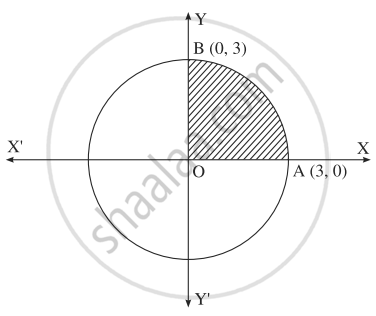# Solve the following : Find the area of the circle x2 + y2 = 9, using integration. - Mathematics and Statistics

Sum

Solve the following :

Find the area of the circle x2 + y2 = 9, using integration.

#### Solution

By the symmetry of the circle, its area is equal to 4 times the area of the region OABO. Clearly for this region, the limits of integration are 0 and 3.From the equation of the circle, y2 = 9 – x2.

In the first quadrant, y > 0

∴ y = sqrt(9 - x^2)

∴ Area of the circle = 4  ......(Area of the region OABO)

= 4int_0^3y.dx = 4int_0^3 sqrt(9 - x^2).dx

= 4[x/2 sqrt(9 - x^2) + (9)/(2) sin^-1 (x/3)]_0^3

= 4[3/2 sqrt(9 - 9) + (9)/(2) sin^-1 (3/3)] - 4[(0)/(2) sqrt(9 - 0) + (9)/(2)sin^1 (0)]

= 4.(9)/(2).(pi)/(2)

= 9π sq.units.

Concept: Application of Definite Integration
Is there an error in this question or solution?
Chapter 5: Application of Definite Integration - Miscellaneous Exercise 5 [Page 190]

#### APPEARS IN

Balbharati Mathematics and Statistics 2 (Arts and Science) 12th Standard HSC Maharashtra State Board
Chapter 5 Application of Definite Integration
Miscellaneous Exercise 5 | Q 2.02 | Page 190
Share